# Basic Math formulas

Find here a comprehensive list of basic math formulas commonly used when doing basic math computation

Average formula:

Let a1,a2,a3,……,an be a set of numbers, average = (a1 + a2 + a3,+……+ an)/n

Fractions formulas: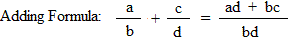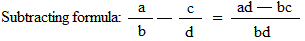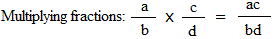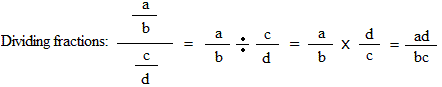Converting an improper fraction to a mixed number: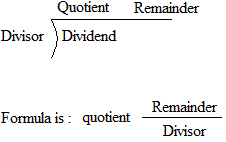Formula for a proportion: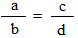In a proportion, the product of the extremes (ad) equal the product of the means(bc),

Thus, ad = bc

Percent:

Percent to fraction: x% = x/100

Percentage formula: Rate/100 = Percentage/base

Rate: The percent.
Base: The amount you are taking the percent of.
Percentage: The answer obtained by multiplying the base by the rate

Consumer math formulas:

Discount = list price × discount rate

Sale price = list price − discount

Discount rate = discount ÷ list price

Sales tax = price of item × tax rate

Interest = principal × rate of interest × time

Tips = cost of meals × tip rate

Commission = cost of service × commission rate

Geometry formulas:

Perimeter:

Perimeter of a square: s + s + s + s
s:length of one side

Perimeter of a rectangle: l + w + l + w
l: length
w: width

Perimeter of a triangle: a + b + c
a, b, and c: lengths of the 3 sides

Area:

Area of a square: s × s
s: length of one side

Area of a rectangle: l × w
l: length
w: width

Area of a triangle: (b × h)/2
b: length of base
h: length of height

Area of a trapezoid: (b1 + b2) × h/2
b1 and b2: parallel sides or the bases
h: length of height

volume:

Volume of a cube: s × s × s
s: length of one side

Volume of a box: l × w × h
l: length
w: width
h: height

Volume of a sphere: (4/3) × pi × r3
pi: 3.14
r: radius of sphere

Volume of a triangular prism: area of triangle × Height = (1/2 base × height) × Height
base: length of the base of the triangle
height: height of the triangle
Height: height of the triangular prism

Volume of a cylinder:pi × r2 × Height
pi: 3.14
r: radius of the circle of the base
Height: height of the cylinder